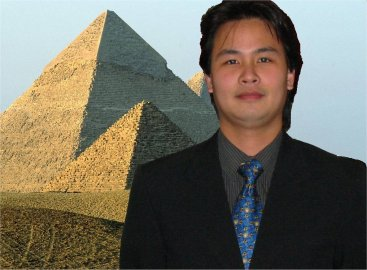# Events

## DMS Algebra Seminar

Time: Nov 01, 2022 (02:30 PM)
Location: ZOOM

Details:Speaker: Tài Huy Hà (Tulane University)

Title: Regularity of graded families of homogeneous ideals.

Abstract: We shall discuss linear bounds and asymptotic values of the regularity of graded families of homogeneous ideals. Let \(S\) be a standard graded algebra over a field \(k\). Let \(I\) be a homogeneous ideal in \(S\). We shall discuss linear bounds for the regularity of \(I^n\), as a function in \(n\), in terms of the generating degrees of \(I\). For a graded family \({I_n}_{n \ge 1}\), we will look at the question of when its asymptotic regularity, defined as the limit of \((reg I_n)/n\) as \(n\) tends to infinity, exists and what it is equal to.